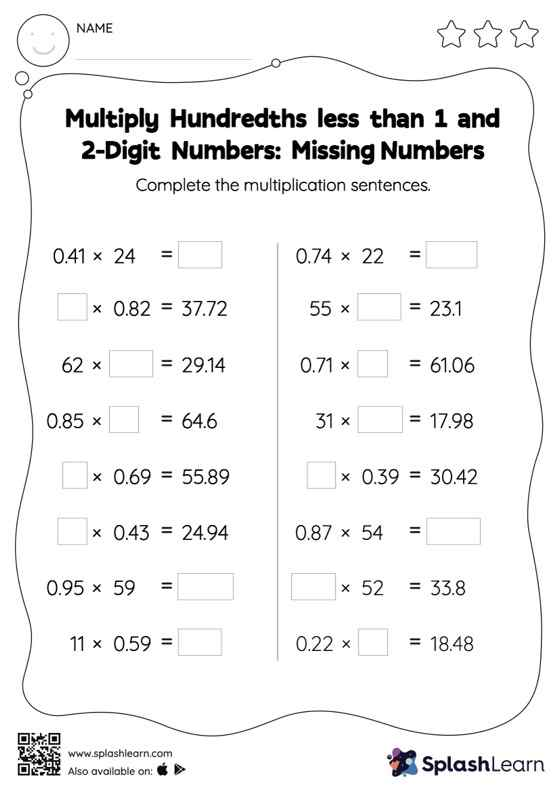# Multiply Hundredths less than 1 and 2-Digit Numbers: Missing Numbers Worksheet

Home > Multiply Hundredths less than 1 and 2-Digit Numbers: Missing NumbersWhen multiplying a decimal by a two-digit number, students can omit the decimal point and add it when they write the answer. Learners have numerous opportunities to put this idea into practice as they find the missing number in multiply hundredths less than 1 and 2-digit numbers worksheet. This worksheet is about practicing with the horizontal format in which numbers are written side by side. To develop flexibility with numbers and operations, students need to have enough practice in this format and not just rely on the vertical/column method.